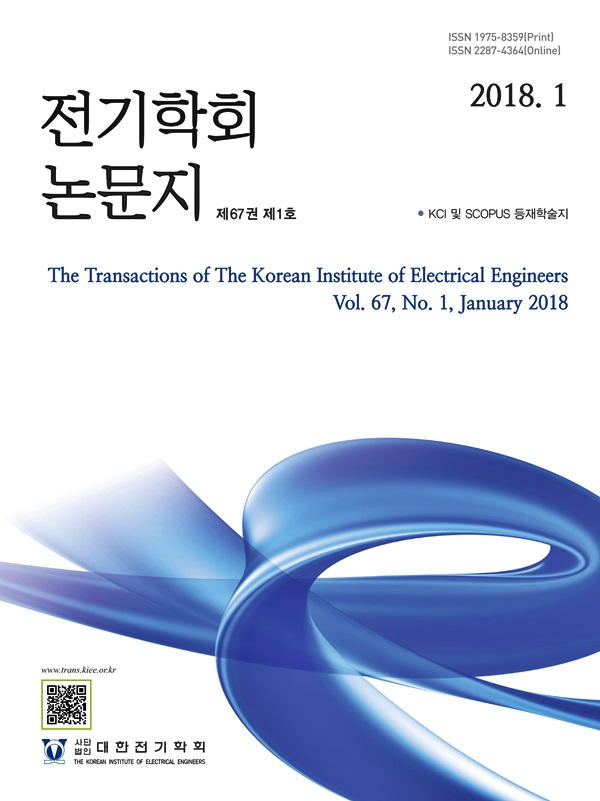Mobile QR Code#### The Transactions of the Korean Institute of Electrical Engineers

##### ISO Journal TitleTrans. Korean. Inst. Elect. Eng.
Title Study of measurement uncertainty evaluation method of three-phase induction motor efficiency 박용준(Yong-Joon Park) ; 허진(Jin Hur) https://doi.org/10.5370/KIEE.2022.71.6.863 pp.863-870 1975-8359 Efficiency; Loss seperation method; Test method; Three-phase Induction motor; Uncertainty In this paper, we propose a method of calculating the measurement uncertainty for the result calculated through the loss separation method, which is one of the efficiency measurement methods of three-phase induction motors. The proposed algorithm calculates the uncertainty for the factors that affect the calculation of each input quantity and then proceeds according to the procedure in Table 2 using a mathematical model. To prove the validity, the efficiency of a 2.2kW three-phase induction motor was measured. The true value of efficiency considering the proposed measurement uncertainties was estimated to be in the range of 88.17 to 88.55%. By the way, considering the tolerance range (1-η)×(-15)% for the reference value 89.5% for measuring the efficiency of the three-phase induction motor, the allowable efficiency reference value is 87.93%. Therefore the estimated efficiency true value should be judged to satisfy the minimum consumption efficiency.